Postfix Evaluation

Infix Expression :

Any expression in the standard form like "2*3-4/5" is an Infix(Inorder) expression.

Postfix Expression :

The Postfix(Postorder) form of the above expression is "23*45/-".

Postfix Evaluation :

In normal algebra we use the infix notation like a+b*c. The corresponding postfix notation is abc*+. The algorithm for the conversion is as follows :
• Scan the `Postfix string` from left to right.
• Initialise an empty stack.
• If the scannned character is an operand, add it to the `stack`. If the scanned character is an operator, there will be atleast two operands in the `stack`.
• If the scanned character is an Operator, then we store the top most element of the stack(`topStack`) in a variable `temp`. Pop the stack. Now evaluate `topStack`(Operator)`temp`. Let the result of this operation be `retVal`. Pop the stack and Push `retVal` into the stack.
Repeat this step till all the characters are scanned.
• After all characters are scanned, we will have only one element in the stack. Return `topStack`.
Example :

Let us see how the above algorithm will be imlemented using an example.

Postfix String : 123*+4-

Initially the Stack is empty. Now, the first three characters scanned are 1,2 and 3, which are operands. Thus they will be pushed into the stack in that order.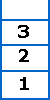Stack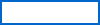Expression

Next character scanned is "*", which is an operator. Thus, we pop the top two elements from the stack and perform the "*" operation with the two operands. The second operand will be the first element that is popped.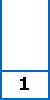Stack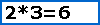Expression

The value of the expression(2*3) that has been evaluated(6) is pushed into the stack.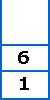StackExpression

Next character scanned is "+", which is an operator. Thus, we pop the top two elements from the stack and perform the "+" operation with the two operands. The second operand will be the first element that is popped.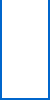Stack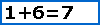Expression

The value of the expression(1+6) that has been evaluated(7) is pushed into the stack.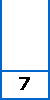StackExpression

Next character scanned is "4", which is added to the stack.StackExpression

Next character scanned is "-", which is an operator. Thus, we pop the top two elements from the stack and perform the "-" operation with the two operands. The second operand will be the first element that is popped.Stack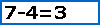Expression

The value of the expression(7-4) that has been evaluated(3) is pushed into the stack.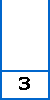StackExpression

Now, since all the characters are scanned, the remaining element in the stack (there will be only one element in the stack) will be returned.

End result :
• Postfix String : 123*+4-
• Result : 3